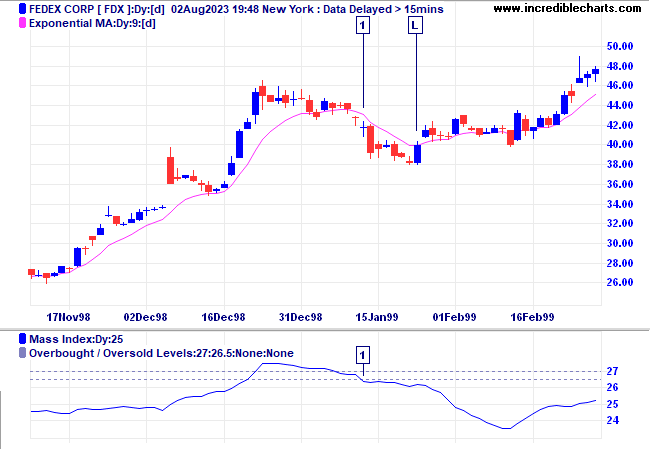# Mass Index Indicator

The Mass Index attempts to predict reversals by comparing the trading range (High minus Low) for each period. Reversals are signaled by a bulge in the index line.

The Mass Index was invented by Donald Dorsey.

The key signal is a reversal bulge. This occurs when the Mass Index (25 period) rises above 27 and falls back below 26.5.

Calculate a 9 day exponential moving average (EMA) of prices:

• Go long if there is a reversal bulge and EMA points downward.
• Go short if there is a reversal bulge and EMA points upward.

Use Trailing Stops to time your entry and Stop Losses on all trades.

### Example

FedEx with   25 day Mass Index, and   9 day exponential moving average.Reversal bulges are very infrequent:

1. Long signal - Mass index completes a reversal bulge at  while the moving average slopes downward. Place a trailing buy-stop above the High of the signal day and move it down above the high of each day until we are stopped in at [L].

## Setup

The default settings are:

• indicator window - 25 days
• upper level - 27.0
• lower level - 26.5

To alter the default settings - Edit Indicator Settings. See Indicator Panel for directions on how to set up an indicator.

## Formula

To calculate the Mass Index:

1. Calculate the range for each period:
High - Low
2. Calculate a 9 day exponential moving average of the range:
EMA [H - L]
3. Calculate a 9 day exponential moving average of the above:
EMA ( EMA [H - L] )
4. Divide the first: EMA by the second:
EMA [H - L] / EMA ( EMA [H - L] )
5. Add the values for the selected number of periods (normally 25).